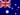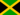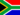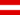# Case study - Depreciation

• Study Reminders

We'll email you at these times to remind you to study

You can set up to 7 reminders per week

#### You're all set

We'll email you at these times to remind you to study

Monday

Tuesday

Wednesday

Thursday

Friday

Saturday

Sunday

• Study Notes
• Discuss This TopicWendy C. 1 0 In the Case study the pizza oven has an estimated life of 6yrs. A straight line approach would probably be best .Anton A. 0 0 What is the balance day adjustment ?Samuel F. 1 0 Depreciation Expense reflects an allocation of an asset's original cost rather than an allocation based on the economic value that is being consumed.Harrison A. 1 0 Case study -Depreciation:it simply requires an exclusive explaination of what depreciation is.However,depreciation represents an expense for a business.The business fixed assets are decreased by a certain value each year snd because the accounting equation must always remain in balance,this decrease must be accounted for somehow. Even though the dollar amount of depreciation is not paid for in cash , the loss in value of the fixed asset must be balanced out and this is done by using two accounts: Deprecition expense and Accumulated Depreciation . The depreciation expense is used to capture the dollar value of dpreciation for an accounting period while Accumulated Depreciation is used to show a running total of how much a fixed asset has depreciated.We take a step furthe by calculating Depreciation. Depreciation is calculated in two main ways but we will take a look at one which is Straight-line depreciation.This method assumes equal amount of depreciation over an asset's useful life. This translates to equal depreciation expense amounts every period . The formula for calculating straight -line depreciation is Cost - saving value divided by useful life. where: Cost = purchase price Useful life = estimated amountof time that the asset will be used by the company. This is some times called service life . Salvage value= estimated amount the asset can be sold for at the end of its useful life.This is some times called residual value. Example: The truck that Ted's trucking purchased for €15000 is expected to be used by the company for 8 years and then sold for €3000. Depreciation is calculated as follows: Depreciation per year= 15000 - 3000 diveded by 8 = €1500.Harrison A. 0 0 What is accelerated depreciation ?Zachary B. 0 0 What are balance day adjustments?Zachary B. 0 0 what arebalanceday adjustments?Zachary B. 0 0 What is depreciation of a case study?Diamond T. 1 0 Depreciation Expense reflects an allocation of an asset's original cost rather than an allocation based on the economic value that is being consumedDouglas R. 1 0 Pizza oven depreciation has a lot of calculations that are examable.
• Text Version